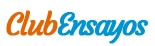# Overview Of The Different Dilution Steps On The MIRA Plus

Enviado por   •  24 de Noviembre de 2011  •  3.240 Palabras (13 Páginas)  •  543 Visitas

Página 1 de 13

Overview of the different dilution steps on the MIRA Plus

1. Dilution of the samples

The dilution of the samples is activated by the REACTION MODES D-R-S, D-R-S-SR1 and D-R-S-SR1-SR2.

The diluent and factor are entered in the parameter setting as following:

A N A L Y S I S

DILUTION NAME : NACL  Diluent (enter name in P62)

FACTOR : 41  Factor

The following dilution factors are available: 4.0 to 150.0

These dilutions need one predilution cuvette.

Pipetted sample volumes to perform these dilutions:

Dilution 1:4.00 ...... 1:150.00

Sample volume (S) 75.0 l 300/factor 2.0 l

Diluent volume (Dil) 15.0 l 90 - S but maximum 50 l * 50.0 l

Reagent volume (R) 210 l 300 - S - Dil * 248 l

Total volume 300 l 300 l * 300 l

* These formulas are theoretical. When the sample volume is a number with more than 1 decimal, it will be rounded to a number with 1 decimal. The diluent and reagent volumes are adapted in order to have the best dilution factor and a total volume in the cuvette that is near to 300 l.

Example: Dilution = 1:41

Theoretical values:

S = 300 / 41= 7.32 l

Dil = 90 - 7.32 = 82.68 l Maximal volume is 50 l ==> Dil = 50 l

R = 300 - 7.32 - 50 = 242.68 l

S = 7.3 l

Total volume in the cuvette = 7.3 x 41 = 299.3

Dil = 90 - 7.3 = 82.7 l Maximal volume is 50 l ==> Dil = 50 l

R = 299.3 - 7.3 - 50 = 242 l

2. Dilution of standards

The dilution of the standards is activated by the REACTION MODES D-R-S, D-R-S-SR1 and D-R-S-SR1-SR2 and the CALIBRATION MODE > SLOPE AVERAGE.

The standards dilution can be performed by 2 different ways:

- Factor: the standards are placed in several cups and they are diluted like the samples.

- Main: the instrument makes several dilutions from a main standard placed in one cup.

2.1 Factor

The diluent and factor are entered in the parameter setting as following:

A N A L Y S I S

DILUTION NAME : NACL  Diluent (enter name in P62)

FACTOR : 41  Factor

TIME: NO

STD: FACTOR

The standards are diluted like the samples (see chapter 1.). They are pipetted from several cups (1 standard / cup).

2.2 Main direct

A N A L Y S I S

DILUTION NAME : NACL  Diluent (enter name in P62)

FACTOR : 41  Factor

TIME: NO

STD: MAIN DIRECT

Main STD: 41 x Ass. value  conc. of the main std.

POS: 1  position of the main std.

FACT.STD - 1: 6 2: 8

3: 12 4: 24

4: 48 6: 96

7: NO 8: NO

The concentration of the standards is automatically calculated by the instrument.

The main direct dilution is performed as below:

Up to 8 standards ca be prepared by diluting a main standard with the factors 1:0, 1:1 and 1:1.5 to 1:30000.

Following conditions must be fulfilled (dilution 1:0 and 1:1 are not taken in account)

- Dfmax / Dfmin  100

- If Dfmin < 9, then Dfmax must be < 150

- If Dfmax > 150, then Dfmin must be > 9 .

Where Dfmin = lowest dilution factor of the serie and

Dfmax = highest dilution factor of the serie

If one dilution is greater than 1:150, an intermediate predilution by 1:6 to 1:200 is performed from the main standard. This intermediate predilution factor (Dfint) is calculated as following:

Dfmin = lowest dilution factor of the serie

Dfmax = highest dilution factor of the serie

Dfint.min = lowest intermediate dilution = Dfmax /150 (If Dfint.min < 6 then Dfint.min = 6)

Dfint.max = highest intermediate dilution = Dfmin /1.5 (If Dfint.max >200 then Dfint.max = 200)

Dfint.max - Dfint.min

Dfint = Dfint.min + ---------------------------- x10

100

All the standards are then prepared from this intermediate predilution. The dilution factors are recalculated by dividing them by the intermediate dilution factor.

Pipetted sample volumes to perform the dilutions that do not request a predilution cuvette:

. Dilution 1:0: the standard is replaced with diluent.

...

Descargar como (para miembros actualizados)  txt (19.4 Kb)
Leer 12 páginas más »
Disponible sólo en Clubensayos.com